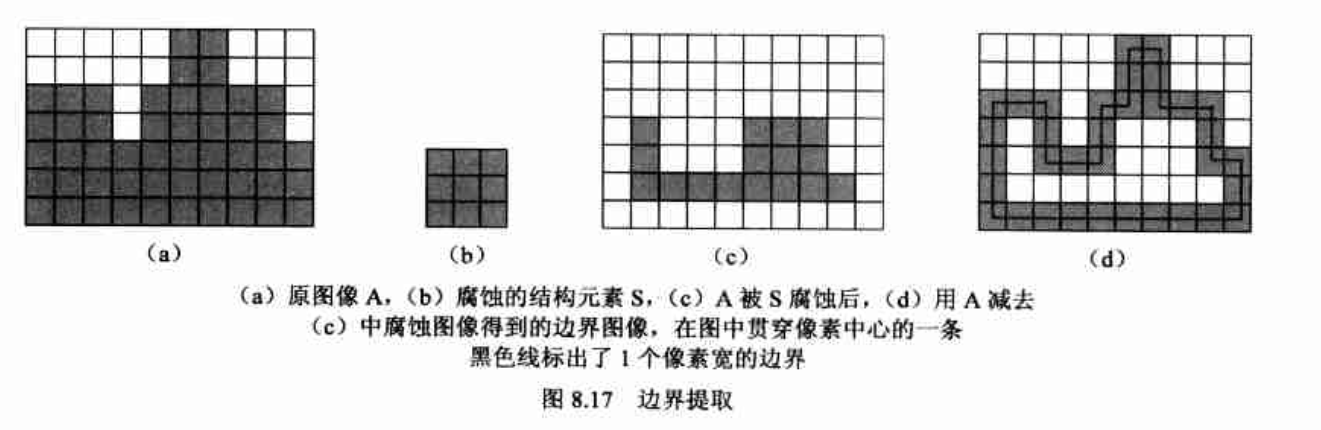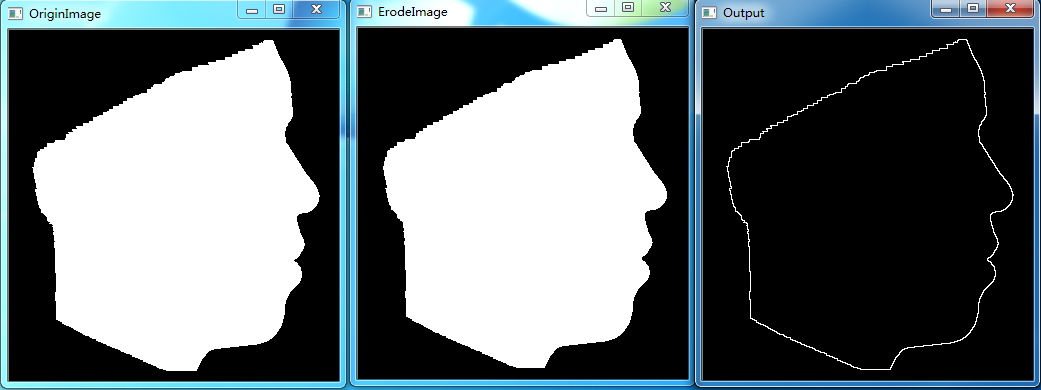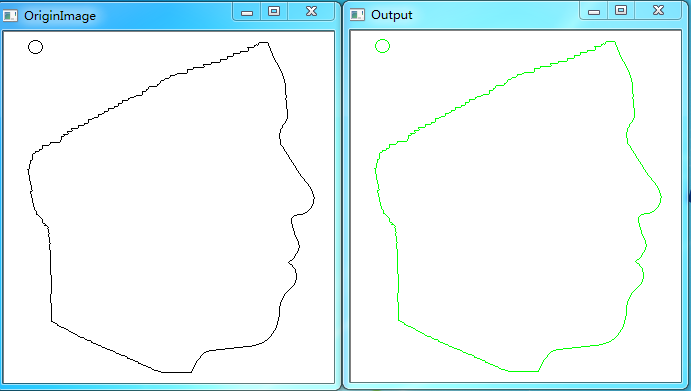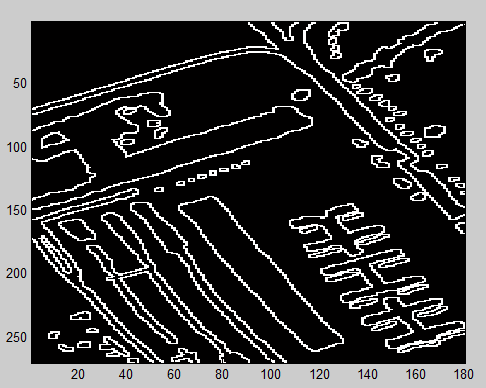2019-08-11 22:07:58 webzhuce 阅读数 509
• ###### 软件测试入门视频教程

软件测试入门视频培训教程：该课程将带你走进“软件测试”的大门，具体内容包括软件测试环境搭建、软件开发模型、产品模型、CMM模型、测试用例、等价类划分、边界值划分、白盒测试、单元测试、bugfree搭建、系统测试、回归测试、验收测试等。本课程以接地气的语言来讲解，让你听的懂，学的会！本课程以全新的方式为你呈现教学内容，清新脱俗独具特色的授课方式将带给你新的体验。

2158232 人正在学习 去看看 李晓鹏

# 边界提取

要在二值图像中提取物体的边界，容易想到的一个方法是将所有物体内部的点删除（置为背景色）。具体地说，可以逐行扫描图像，如果发现一个黑点的8个邻域都是黑点，则该点为内部点，在目标图 像中将它删除。实际上这相当于采用一个3*3的结构元素对原图进行腐蚀，使得只有那些8个邻域都有黑点的内部点被保留，再用原图像减去腐蚀后的图像，恰好删除了这些内部点，留下了边界像素。这过程如下图所示。## 示例演示

利用OpenCV实现上面边界提取的功能。

``````#include<opencv2/opencv.hpp>
using namespace cv;

int main(int argc, char *argv[])
{
Mat element = getStructuringElement(MORPH_RECT, Size(3, 3));
imshow("OriginImage", originimage);
Mat erodeimage;
cv::erode(originimage, erodeimage, element);
imshow("ErodeImage", erodeimage);

Mat output = originimage -  erodeimage;
imshow("Output", output);

waitKey(0);
return 0;

}
``````

## 运行结果# 边界跟踪

为了依次记录边界上的每个像素，边界跟踪首先按照某种扫描规则找到目标物体边界上的一个像素，然后就以该像素为起始点，根据某种顺序（如顺时针或逆时针）依次找出物体边界上的其余像素，直到又回到起始点，完成整条边界的跟踪。

## 示例演示

在一幅图像中，实现跟踪多个边界的功能。对于带孔洞的物体也可以跟踪至其孔洞的轮廓。

``````#include<opencv2/opencv.hpp>
using namespace cv;

//only process binary image
//black is boundary
std::vector<std::vector<cv::Point>> TraceBoundary(Mat &image)
{
std::vector<std::vector<Point>> boundaryset;
Point start, current, next; // start point and current point
//search dirction array
int direction ={{-1, 1}, //left-down
{0, 1}, // down
{1, 1}, //right-down
{1, 0}, //right
{1, -1}, //right-up
{0, -1}, //up
{-1, -1}, //left-up
{-1, 0}  // left
};
int begindirection = 0, currentdirection = 0;
bool atstart = false, findboundary = false;

for(int i = 0; i < image.rows; i++)
{
for(int j = 0; j < image.cols; j++)
{
if(image.at<uchar>(i, j) == 0) //find start point
{
start.x = j;
start.y = i;
current = start;
atstart = true;
findboundary =  true;
std::vector<Point> points;
points.push_back(current);
std::cout << "Start: " << j << " " << i << std::endl;
while((current.x != start.x) || (current.y != start.y) || atstart)
{
atstart = false;

//search next point
next.x = current.x + direction[currentdirection];
next.y = current.y + direction[currentdirection];
int searchtimes = 1;
while(next.x < 0 || next.x >= image.cols || next.y < 0 || next.y >= image.rows || image.at<uchar>(next) == 255)
{
currentdirection++; //rotate 45 degrees counterclockwise
currentdirection %= 8;
next.x = current.x + direction[currentdirection];
next.y = current.y + direction[currentdirection];
//there are no boundary points in 8 domains, which means they are isolated points
if(++searchtimes >= 8)
break;
}
if(image.at<uchar>(next) == 0) // find next point
{
std::cout << "Next: " << next.x << " " << next.y << std::endl;
points.push_back(next);
current = next;
currentdirection -= 2;
if(currentdirection < 0)
currentdirection += 8;

}
else // not find next point
{
findboundary = false;
break;
}
}
if(findboundary)
{
boundaryset.push_back(points);
for(auto &p : points)
{
image.at<uchar>(p) = 255;
}
}
} // find boundary one time
} // for j
} // for i
return boundaryset;
}

int main(int argc, char *argv[])
{
imshow("OriginImage", originimage);

Mat image;
cvtColor(originimage, image, CV_BGR2GRAY);
std::vector<std::vector<Point>> boundaryset = TraceBoundary(image);

//show result
Mat result;
originimage.copyTo(result);
for(auto &points : boundaryset)
{
for(auto &p : points)
{
result.at<Vec3b>(p)= 0;
result.at<Vec3b>(p)= 0;
result.at<Vec3b>(p)= 255;
}
}
imshow("Output", result);

waitKey(0);
return 0;

}
``````

## 运行结果2018-05-02 16:53:04 qq_37241117 阅读数 3537
• ###### 软件测试入门视频教程

软件测试入门视频培训教程：该课程将带你走进“软件测试”的大门，具体内容包括软件测试环境搭建、软件开发模型、产品模型、CMM模型、测试用例、等价类划分、边界值划分、白盒测试、单元测试、bugfree搭建、系统测试、回归测试、验收测试等。本课程以接地气的语言来讲解，让你听的懂，学的会！本课程以全新的方式为你呈现教学内容，清新脱俗独具特色的授课方式将带给你新的体验。

2158232 人正在学习 去看看 李晓鹏

%%%一下是matlab代码

%%%TureFlag是我的类别标签图，本来有7类地物，二值化一下就可以。所以如果你的也不是二值图，你可以将图像二值化再做

function [TrueFlag]=BoundaryJudge(Image,TrueFlag)

[m,n]=size(TrueFlag);
imgn=zeros(m,n);
ed=[-1 -1;0 -1;1 -1;1 0;1 1;0 1;-1 1;-1 0];
for i=2:m-1
for j=2:n-1
if TrueFlag(i,j)==1%如果当前像素是前景像素
for k=1:8
ii=i+ed(k,1);
jj=j+ed(k,2);
if TrueFlag(ii,jj)==0%当前像素周围如果是背景，边界标记图像相应像素标记
imgn(ii,jj)=1;
end
end
end
end

end

figure;                       %由于只有0,1，所以colormap(gray)也没关系，显示出来就是黑白图
imagesc(imgn);
colormap(gray);

end2015-12-25 16:15:44 liu_xiao_cheng 阅读数 6215
• ###### 软件测试入门视频教程

软件测试入门视频培训教程：该课程将带你走进“软件测试”的大门，具体内容包括软件测试环境搭建、软件开发模型、产品模型、CMM模型、测试用例、等价类划分、边界值划分、白盒测试、单元测试、bugfree搭建、系统测试、回归测试、验收测试等。本课程以接地气的语言来讲解，让你听的懂，学的会！本课程以全新的方式为你呈现教学内容，清新脱俗独具特色的授课方式将带给你新的体验。

2158232 人正在学习 去看看 李晓鹏
形态学一般是使用二值图像，进行边界提取，骨架提取，孔洞填充，角点提取，图像重建。

基本的算法:膨胀腐蚀，开操作，闭操作，击中击不中变换

边界提取主要涉及腐蚀和求补集操作int  picProcessBasics::IMGEdgeExtracting(IplImage* pImg,IplImage* pDestImg,double threshold,int pos)
{
if(NULL == pImg || NULL == pDestImg)
return -1;
if(pDestImg->nChannels!=1 )
{
cout<<"It's not gray image!"<<endl;
return -1;
}

IplImage* tempImage= cvCreateImage(cvGetSize(pImg), 8, 1);
if(pImg->nChannels != 1)
{
cvCvtColor(pImg,tempImage,CV_RGB2GRAY);
}
else
cvCopy(pImg,tempImage,0);

//转换成二值图像
cvThreshold(tempImage, pDestImg, threshold, 255, CV_THRESH_BINARY);

IplConvKernel *element = cvCreateStructuringElementEx( pos*2+1, pos*2+1, pos, pos, CV_SHAPE_RECT, 0 );
cvErode( tempImage, tempImage, element, 1); // 侵蚀,磨蚀
cvReleaseStructuringElement( &element );

for(int i = 0; i < tempImage->height; i++){
for(int j = 0; j < tempImage->width; j++){

pDestImg->imageData[pDestImg->widthStep * i + j ]=pDestImg->imageData[pDestImg->widthStep * i + j ] - tempImage->imageData[tempImage->widthStep * i + j ];
}
}
cvReleaseImage(&tempImage);

return 0;
}﻿﻿

2017-08-07 17:36:14 ling_xiobai 阅读数 38551
• ###### 软件测试入门视频教程

软件测试入门视频培训教程：该课程将带你走进“软件测试”的大门，具体内容包括软件测试环境搭建、软件开发模型、产品模型、CMM模型、测试用例、等价类划分、边界值划分、白盒测试、单元测试、bugfree搭建、系统测试、回归测试、验收测试等。本课程以接地气的语言来讲解，让你听的懂，学的会！本课程以全新的方式为你呈现教学内容，清新脱俗独具特色的授课方式将带给你新的体验。

2158232 人正在学习 去看看 李晓鹏``````img = imread('图形.jpg');
figure,imshow(img);
img = im2bw(img);%（图像分割）转化为二值图
img = not(img);%把图像想表达的内容变成1
figure,imshow(img);``````bwboundaries函数可以追踪目标的外边界，以及这些目标中孔的边界。它返回：
1.一个元胞数组（B）（补充：元胞数组不同于普通数组，普通数组存放的元素都是一样的数据结构，但元胞数组存储的可以是不同的数据类型，所以元素间不相关）
2.返回一个标号矩阵L，其中每个目标赋予一个标号（下面有示例）
3.返回总目标数N等

``````[B,L] = bwboundaries(img);
figure,imshow(img);
hold on;
for k = 1:length(B)
boundary = B{k};
plot(boundary(:,2),boundary(:,1),'g','LineWidth',2);
end````````````[L,N] = bwlabel(img);
img_rgb = label2rgb(L,'hsv',[.5 .5 .5],'shuffle');
figure,imshow(img_rgb);hold on
for k =1:length(B)
boundary = B{k};
plot(boundary(:,2),boundary(:,1),'w','LineWidth',2);
text(boundary(1,2)-11,boundary(1,1)+11,num2str(k),'Color','y','Fontsize',14,'FontWeight','bold');
end````````````stats = regionprops(L,'all');%统计的数保留在stats内
temp = zeros(1,N);
for k = 1:N
%计算thinness ratio（细度比例）
temp(k) = 4 * pi * stats(k,1).Area / (stats(k,1).Perimeter)^2;
stats(k,1).ThinnessRatio = temp(k);
%计算aspect ratio
temp(k) = (stats(k,1).BoundingBox(3))/(stats(k,1).BoundingBox(4));
stats(k,1).AspectRatio = temp(k);
end````````````areas = zeros(1,N);
for k = 1:N
areas(k) = stats(k).Area;
end
TR = zeros(1,N);

for k = 1: N
TR(k) = stats(k).ThinnessRatio;
end
cmap = colormap(lines(21));
figure
for k = 1:N
scatter(areas(k),TR(k),[],cmap(k,:),'filled');
ylabel('Thinness Ratio'),xlabel('Area');
hold on
end``````OK，这次学习就到这里，希望大家多多交流，一起学习，分享知识是一种美德，谢谢观看。

2019-09-04 21:30:24 lengo 阅读数 809
• ###### 软件测试入门视频教程

软件测试入门视频培训教程：该课程将带你走进“软件测试”的大门，具体内容包括软件测试环境搭建、软件开发模型、产品模型、CMM模型、测试用例、等价类划分、边界值划分、白盒测试、单元测试、bugfree搭建、系统测试、回归测试、验收测试等。本课程以接地气的语言来讲解，让你听的懂，学的会！本课程以全新的方式为你呈现教学内容，清新脱俗独具特色的授课方式将带给你新的体验。

2158232 人正在学习 去看看 李晓鹏
``````clc;
clear all;
close all;

%读入图像，并转换为double型，该计算针对的是二值图像，所以加入图像时需注意
%膨胀测试图像
%获得图像大小
[M,N]=size(I);
%存放膨胀后的图像
J=zeros(M,N);
%===============================边界提取===================================
%结构元素
n=3;
B=ones(n,n);
n_B=length(find(B==1));
%这里需要B对其原点进行翻转，因为B是对称的，所以翻转后的结果与其本身相同
l_m=floor(n/2);
l_n=floor(n/2);
%腐蚀操作
for x=1:M
for y=1:N
%从扩展图像中取出子图像
%将结构元素与子图像点乘,即逻辑“与”操作
c=B.*Block;
%比较结构元素与c中的1的数量，如果一样多，则该点的值为1
ind=find(c==1);
if length(ind)==n_B
J(x,y)=1;
end
end
end
Beta=I-J;
imshow(Beta,[]);``````